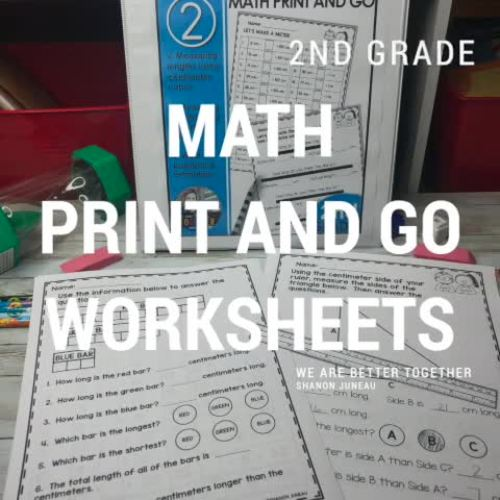# 2nd Grade Measurement Worksheets | Measurement Word Problems

Rated 4.62 out of 5, based on 21 reviews
21 Ratings;
2nd, Homeschool
Subjects
Resource Type
Standards
Formats Included
• PDF
• Internet Activities
Pages
48 pages
\$4.00
List Price:
\$5.00
You Save:
\$1.00
\$4.00
List Price:
\$5.00
You Save:
\$1.00
Report this resource to TPT
Easel Activity Included
This resource includes a ready-to-use interactive activity students can complete on any device.  Easel by TPT is free to use! Learn more.
Compatible with Digital Devices
The Teacher-Author has indicated that this resource can be used for device-based learning.

#### What educators are saying

This has been very useful in finding other ways for scholars to practice what they are learning. It is easy to use for me and my students.
##### Also included in
1. This amazing year-long bundle has everything you need to make sure your students are grasping the 2nd grade concepts they need to be mathematicians! These cover all of the standards.SAVE 20% WITH THIS YEAR LONG BUNDLE!Here's what's in the resource:Module 1: Make a ten and adding to tenMake the next
Price \$32.00Original Price \$53.50Save \$21.50

### Description

These 2nd Grade Measurement math worksheets are great to teach/reinforce measurement using centimeter cubes, rulers, estimation, and relating addition and subtraction to length. These 2nd grade math worksheets can be used to introduce a concept, review, assessment, homework, or center practice.

BUNDLED FOR CONVENIENCE!

These worksheets cover:

• Measurement using centimeter cubes
• Measurement using a centimeter ruler
• Estimation
• Relate addition and subtraction to length
• Solving addition and subtraction word problems using cm and m

Main Standards Covered:

• 2.MD.1 Measure the length of an object by selecting and using appropriate tools such as rulers, yardsticks, meter sticks, and measuring tapes.
• 2.MD.2 Measure the length of an object twice, using length units of different lengths for the two measurements; describe how the two measurements relate to the size of the unit chosen.
• 2.MD.3 Estimate lengths using units of inches, feet, centimeters, and meters.
• 2.MD.4 Measure to determine how much longer one object is than another, expressing the length difference in terms of a standard length unit.
• 2.MD.5 Use addition and subtraction within 100 to solve word problems involving lengths that are given in the same units, e.g., by using drawings (such as drawings of rulers) and equations with a symbol for the unknown number to represent the problem.
• 2.MD.6 Represent whole numbers as lengths from 0 on a number line diagram with equally spaced points corresponding to the numbers 0, 1, 2, …, and represent whole-number sums and differences within 100 on a number line diagram.

WHO LIKES A DEAL?

• Follow me and be notified when new products are uploaded. New products are always 50% off for the first 24 hours they are posted (except for resources that are under \$1.50). It pays to follow me!

QUESTIONS OR CONCERNS

I sure appreciate you!

Shanon Juneau ❤️

We Are Better Together

Total Pages
48 pages
N/A
Teaching Duration
N/A
Report this resource to TPT
Reported resources will be reviewed by our team. Report this resource to let us know if this resource violates TPT’s content guidelines.

### Standards

to see state-specific standards (only available in the US).
Measure the length of an object by selecting and using appropriate tools such as rulers, yardsticks, meter sticks, and measuring tapes.
Estimate lengths using units of inches, feet, centimeters, and meters.
Measure to determine how much longer one object is than another, expressing the length difference in terms of a standard length unit.
Use addition and subtraction within 100 to solve word problems involving lengths that are given in the same units, e.g., by using drawings (such as drawings of rulers) and equations with a symbol for the unknown number to represent the problem.
Represent whole numbers as lengths from 0 on a number line diagram with equally spaced points corresponding to the numbers 0, 1, 2,..., and represent whole-number sums and differences within 100 on a number line diagram.